Related Articles

# Matlab – Matrix

• Last Updated : 21 Jun, 2021

A Matrix is a two-dimensional array of elements. In MATLAB, the matrix is created by assigning the array elements that are delimited by spaces or commas and  using semicolons to mark the end of each row. Now let’s have a glance at some examples to understand it better.

Syntax:

a = [elements; elements]

Example: Creating a Matrix

## MATLAB

 `% MATLAB program to create``% a matrix` `%Number Matrix``x = [1 2 3;4 5 6;7 8 9]` `%String Matrix``y = [``'Geeks'``;``'Geeks'``]`

Output: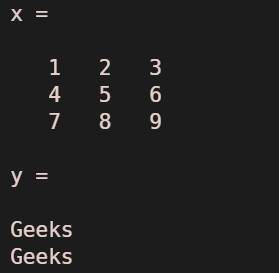Example: Knowing the size of the Matrix

## MATLAB

 `% MATLAB program to know``% the size of a matrix` `% Creating matrices``x = [1 2 3 4;4 5 6 7;7 8 9 10];``xSize = size(x)` `y=[``'Geeks'``;``'Geeks'``];``ySize = size(y)`

Output: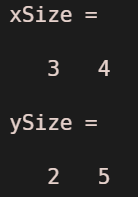### Accessing the elements of a matrix

To reference an element in a matrix, we write matrix(m, n). Here m ande n are row and column indexes.

Example 1:

## MATLAB

 `% MATLAB program to access``% a particular element` `% Creating matrices``x = [1 2 3 4;4 5 6 7;7 8 9 10];``x(3,2)` `y=[``'Geeks'``;``'Geeks'``];``y(1,2)`

Output: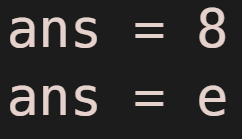To access multiple elements in the matrix, we write

matrix(x:y;,xy)

Example 2:

## MATLAB

 `% MATLAB program to access``% a multiple elements` `% Creating matrices``x = [1 2 3 4;4 5 6 7;7 8 9 10];` `% Accessing all rows and columns``x(:,:)` `% Accessing all the elements``% in the first two rows``x(1:2,:)` `% Accessing all the elements``% from 2nd to last element``% in every row.``x(:,2:``end``)` `% Accessing elements from 2nd index``% to 3rd index in the first two columns``x(1:2,2:3)`

Output: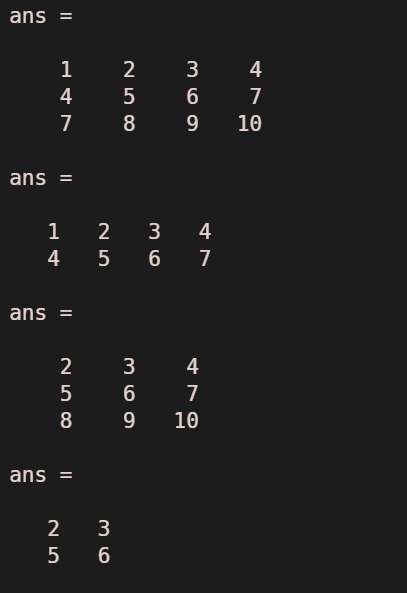### Adding elements/dimensions to a matrix

To add elements/dimension to a matrix we can use one of the following methods:

cat(dimension,A,B,….)

or

x = [A;B]

In the above syntax, A & B are matrices that we need to pass into the function cat() or concatenate by using square brackets. Here dimension parameter must be either 1 or 2 for table or timetable input.

Example 1:

## MATLAB

 `% MATLAB program to add``% dimensions to a matrix` `% Creating matrices``x = [1 2 3 4;4 5 6 7];``y = [7 8 9 10;11 12 13 14];` `% Concatenating two matrices``% or adding a row``a = [x;y]``b = [x;11 12 13 14]` `%Adding a column to a matrix``x(:,5)=[15 16]`

Output: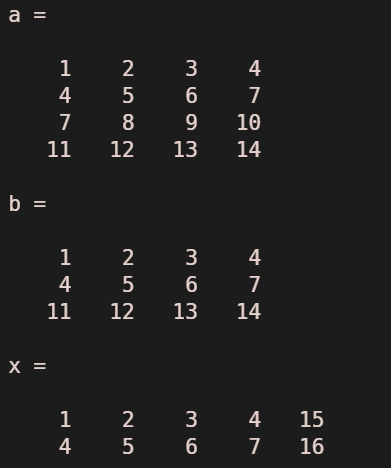Example 2:

## MATLAB

 `% Matlab Program to``% explain concatenation` `x = [1 2 3 4;4 5 6 7];` `% Creating a new matrix by``% concatenating multiple rows``% of a matrix``a = x([1,2,2,1],:)``  ` `% Creating a new matrix by``% concatenating multiple columns``% of a matrix``b = x(:,[1,3,2])`

Output: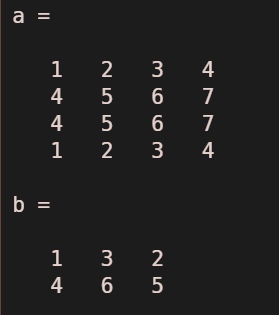Example 3:

## MATLAB

 `% MATLAB program to concatenate``% two matrices using cat() function` `% Creating matrices``x = [1 2 3 4;4 5 6 7];``y = [1 2 3 4;4 5 6 7];` `% Concatenating two matrices``% Using cat() function``a = cat(1,x,y)``b = cat(2,x,y)`

Output: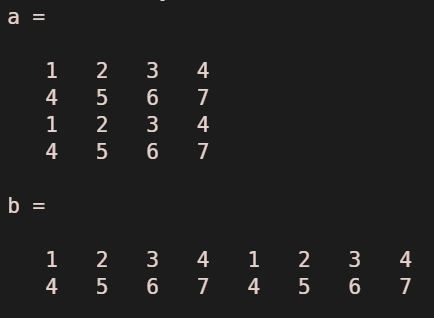Example 4: Deleting a row/column of a matrix

## MATLAB

 `% MATLAB program to delete``% a row or a column` `% Creating matrices``x = [1 2 3 4;4 5 6 7;7 8 9 10];` `% Deleting 3rd row of``% a matrix``x(3,:) = []` `% Deleting 3rd column in``% every row of a matrix``x(:,3) = []`

Output: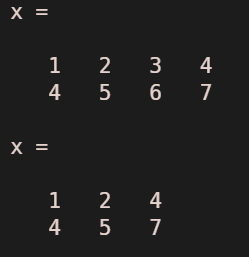Note:

• Unlike most of the programming languages, MATLAB array indexes start from 1.
• To perform operations(almost all) on the matrices, dimensions must be the same.
• A semicolon must be added at each end of the statement in order to avoid multiple outputs.
• In MATLAB, a string is a character array.
• In MATLAB, length() gives the length of an array, and size() gives the size of a matrix.

My Personal Notes arrow_drop_up